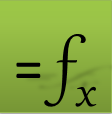# 3D Interpolation in Excel

## =INTERPXYZ(xy, z, q, [options])

INTERPXYZ is a powerful 3D interpolation function based on natural neighbor algorithm. It can be used to probe the value of a surface passing through a set of scattred data points at arbitrary points.

Use INTERPXYZ to interpolate a set of scattered (x,y,z) data points at arbitrary (xq,yq) points.

INTERPXYZ automatically sorts your data set and averages the z values if your data set contains duplicate (x,y) points.

### Required Inputs

xy a 2-column array of scattered (x,y) points coordinates.

z a vector of corresponding z values

q a two-column array of one or more (xq,yq) point(s) to interpolate at.

If q is a vector of points, run INTERPXYZ as an array formula.

### Optional Inputs

ctrl a set of key/value pairs for algorithmic control as detailed below.

Description of key/value pairs for algorithmic control

 Key PERTURB Admissible Values (Boolean) True or False Default Value True Remarks A True value will slightly perturb the locations of the scattered (x,y) points to aid stability of Voronoi construction. This perturbation has no significant effect on the accuracy of the interpolated data.

 Key NONNEGZ Admissible Values (Boolean) True or False Default Value False Remarks Set to True to force negative interpolated z values to zero.This may be useful to mask roundoff errors during interpolation when the surface passing through the points is known to be nonnegative.

INTERPXYZ implements the natural neighbor interpolation algorithm.

Question or Comment? Email us:
support @ excel-works.comExceLab: Transforming Excel into a Calculus Power House

ExceLab functions and methods are protected by USA Patents 10628634, 10114812, 9892108 and 9286286.